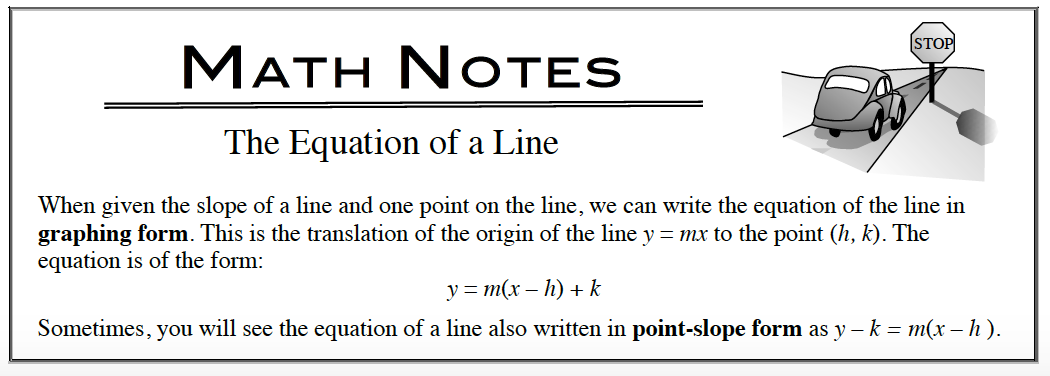### Home > APCALC > Chapter 1 > Lesson 1.1.1 > Problem1-8

1-8.

Write the equation of the line through the point $(–5, –2)$ with a slope of $–3$ in graphing form using the method developed in problem 1-7. Refer to the Math Notes box for help. Homework Help ✎Leave your answer in Point-Slope Form. There is no reason (in this case) to convert this equation into $y$-intercept form.